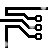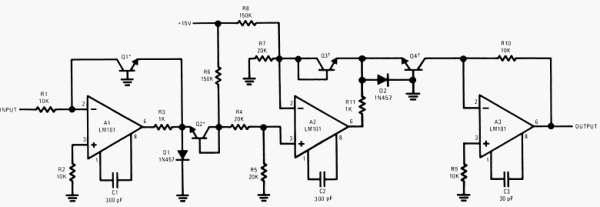## Root Extractor

### By Robert J. Widlar

Taking the root of a number using log converters is a fairly simple matter. All that is needed is to take the log of a voltage, divide it by, say 1⁄2 for the square root, and then take the antilog.

Taking the root of a number using log converters is a fairly simple matter. All that is needed is to take the log of a voltage, divide it by, say 1⁄2 for the square root, and then take the antilog. A circuit which accomplishes this is shown in Figure 1. A1 and Q1 form the log converter for the input signal. This feeds Q2 which produces a level shift to give zero voltage into the R4, R5 divider for a 1V input. This divider reduces the log voltage by the ratio for the root desired and drives the buffer amplifier, A2. A2 has a second level shifting diode, Q3, its feedback network which gives the output voltage needed to get a 1V output from the antilog generator, consisting of A3 and Q4, with a unity input. The offset voltages of the transistors are nulled out by imbalancing R6 and R8 to give 1V output for 1V input, since any root of one is one.Figure 11. Root Extractor

Note: *The “extraction” used here doubtless has origin in the dental operation most of us would fear less than having to find even a square root without tables or other aids.

Q2 and Q3 are connected as diodes in order to simplify the circuitry. This doesn’t introduce problems because both operate over a very limited current range, and it is really only required that they match. R7 is a gain-compensating resistor which keeps the currents in Q2 and Q3 equal with changes in signal level.

As with the multiplier/divider, the circuit is insensitive to temperature as long as all the transistors are at the same temperature. Using transistor pairs and matching them as shown minimizes the effects of gradients.

The circuit has 1-percent accuracy for input voltages between 0.5 and 50V. For lower input voltages, A1 and A3 must have their offsets balanced out individually.206009 December 2007